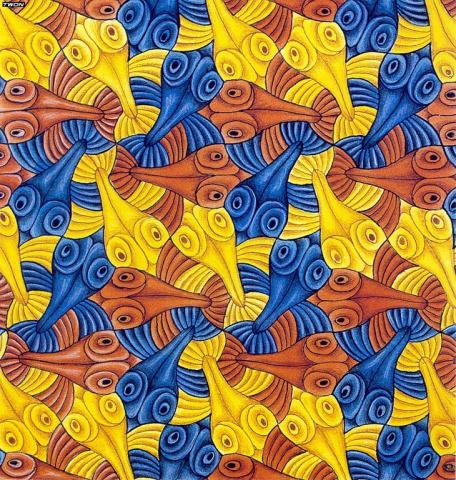# SC 2019 Semilab: Geometries and Symmetriesby Misha Lyubich

Why is it possible to construct with the compass and ruler a regular 5-gon but is impossible to construct the cube of volume 2? Why there is a formula solving a quadratic equation but there is no such a formula for an equation of degree five? Why it took physicists by surprise to discover a crystal that appeared to have a rotational 72 degrees symmetry?
And what all this has to do with elementary particles and Escher's paintings?

The notion that unifies all these themes is a group of symmetries".

In our semilab we will introduce this notion and will illustrate it with a variety of geometric themes (in the spirit of the Klein Erlangen Program" asserting that there are many geometries corresponding to various symmetry groups). We will start with a familiar group of Euclidean motions of the plane (translations and rotations) and will describe associated wallpapers. Then we will add to it similarities and Mobius maps" that can change the size of figures but respect angles (which is useful for cartography). Nice fractal patterns, like Apollonian gaskets", appear in this kind of geometry. We will move on to spherical and hyperbolic geometries, challenging the well known to you fact that the sum of angles of a triangle is equal to 180 degrees. Five Platonic bodies and infinitely many double bagels" (called krendel's" in Russian) will be served for desert.
We will finish with a brief introduction to the projective geometry where you cannot distinguish an ellipse from a hyperbola and where parallel lines cross: indeed, two rails of a train track meet at the horizon...

Back to the list of semilabs